# Variable Reference and Instance of a Class in C#

## Differences Between Variable, Reference, and Instance of a Class in C#

In this article, I am going to discuss the differences between Variables, References, and Instances of a Class in C# with Examples. Please read our previous article where we discussed Static Class in C# with Examples. As a programmer, it is very important for us to understand what are Variables, References, and Instances of a Class in C#.

##### Differences Between Variable, Reference, and Instance of a Class in C#
1. Variable of a Class: A copy of the class that is not initialized.
2. Instance of a Class: A copy of the class that is initialized by using the new keyword which has its own memory and is never shared with another instance.
3. Reference of a Class: A copy of a class that is initialized by using an existing instance and references of a class will not have any memory allocation, they will be sharing the memory of the same instance that is assigned for initializing the variable.

Let us understand the above definitions in detail with examples.

##### What is a Class?

Simply speaking a class is a user-defined type in C#. We are saying string is a data type. But this string data type is created as a class in C#. It’s a pre-defined class and all pre-defined classes or user-defined classes in C# are also called data types.

The second point that you need to understand is that we can never consume a class directly. How to consume a class means we need to create a copy of that class. Why create a copy of the class? Let us understand this. We know int is a data type in C#. Can we use the int data type as follows?
int = 10;

No. It’s not possible. We can never consume int like the above. Why because int is only a blueprint for your particular data, it does not have memory allocation for your data. If you want memory allocation for your data, then you should like as follows:
int I = 10;

What is “I” here? Here, “I” is a copy of the int data type. In the same way, the following statement is also invalid.
string = “Hello”;
Here, the string is a class and a class is a user-defined data type. You cannot consume it directly. If you want to consume string, then you need to use it as follows.
string s = “Hello”;

What is s here? Here, s is a copy of the string data type.
See, to build a house we require a plan. Can we build a house without a plan? No. Not possible. So, every house requires a plan. The same thing is also here. Here, int and string are nothing but the plan of your house and i and s are your constructed houses. To build a house, you will consult with an architect. The architect will give you the plan? Can you leave in the plan? No, you cannot live in the plan. So, if you really want to live, then you need a house that is constructed based on the plan.

Exactly the same thing also happens here. Here, int is the plan and i is the constructed house based on the plan. So, now, the value 10 is nothing but you, living in a constructed house which is in our example i, and the house is constructed based on the plan i.e. int. So, the point that you need to remember is that the data type is always going to be the plan and a copy of that data type is the implementation of that plan.

Note: Every class whether it is predefined or user-defined, is going to be a data type. To consume the class what we need to do is we need to create a copy of that class. Until and unless we are not creating a copy of that class, the memory will not be allocated. If you want the memory to be allocated, then you need to create a copy of the type.

##### Example to Understand How to Consume a Type in C#:

Please have a look at the following example. Here, I have created an integer variable called x with the value 10, and then within the Main method and I am just printing the x value in the console window.

```using System;
namespace VariablesDemo
{
internal class Example
{
int x = 10;
static void Main(string[] args)
{
Console.WriteLine(x);
}
}
}
```

Now, if you try to run the above code, you will get the following error. Here, we are getting the error when we try to print the value of x. This is because x is an instance member or you can say non-static member and you cannot access any non-static member directly from a static block, and here the Main method is a static block.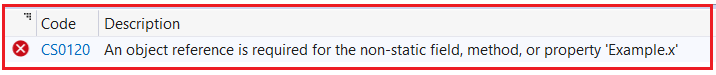Now, the Main method is a static block and x is an instance member, so you cannot access x directly. If you want to access x, then first you need to create an instance of the Example class or a copy of the Example class and using the Example class instance only you can access the value of x as shown in the below example.

```using System;
namespace VariablesDemo
{
internal class Example
{
int x = 10;
static void Main(string[] args)
{
Example e = new Example();
Console.WriteLine(e.x);
}
}
}
```

So, here e is an instance of the Example class, or you can say e is a copy of the Example class. And now, the memory is allocated for that instance e. An instance of a class is created by using the new keyword only.

So, the point that you need to remember is we can only print the value of x by using the instance of the class or copy of the class and the instance is created by using the new keyword only in C#.

##### Variables of a Class in C#:

Now, let us understand what is a variable of a class in C#. Please observe the following code.

Example e;

Here, we are not initializing e. So, what is a variable of a class? Variable of a class means a copy of a class that is not initialized. It is also considered an uninitialized copy of a class. For a better understanding, please have a look at the following code.

```using System;
namespace VariablesDemo
{
internal class Example
{
int x = 10;
static void Main(string[] args)
{
//Variable
//Uninitialized copy of class Example
Example e;
Console.WriteLine(e.x);
}
}
}
```

Now, if you try to run the above code, you will get the following compile time error i.e. Use of unassigned local variable ‘e’. Here, e is not an instance, you can see in the error message, it is saying local variable e. So, here e is a local variable. And you are trying to access a member called x using a variable which is not possible in C# language and hence you are getting the following error.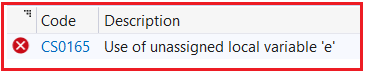So, the point that you need to remember is you can access the non-static members of a class by using instance only, not by using the variables. An instance of a class is created by using the new keyword only. And if the new keyword is not used, means it is not an instance, it is just a variable. Now, observe the following code.

Example e; Here e is a variable of class Example

e = new Example(); Now, here, e is an instance of class Example. As soon as you use the new keyword, e becomes an instance and now, you can access the x variable using the e instance as shown in the below example.

```using System;
namespace VariablesDemo
{
internal class Example
{
int x = 10;
static void Main(string[] args)
{
Example e; //e is Variable of class Example
e = new Example(); //e is instance of class Example
Console.WriteLine(e.x);
}
}
}
```

So, we can create an instance in C# using the following two ways and both are going to be the same. In the first case, we have done the declaration and initialization at the same line. And in the second we have separated the declaration and initialization into two lines. First, we declare the variable and then we initialize the object. When we initialize the variable, then the variable becomes an instance of the class and using the instance we can access only the non-static members of the class.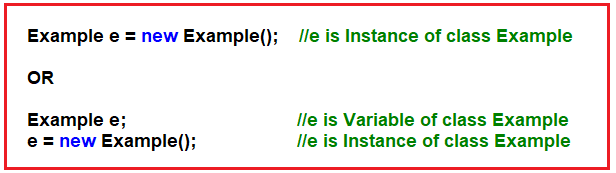##### Difference Between Variable and Instance of a Class in C#:

Variables of a Class are nothing but the uninitialized copy of a class. They don’t occupy any memory. They are holding null. And using variables, we cannot consume the member of a class. When we initialize a variable with the new keyword, then it becomes an instance. An instance is nothing but a copy of a class. It occupies memory and using instances of a class, we can access the non-static members of that class. For a better understanding of the difference between Variable and Instance in C#, please have a look at the following diagram.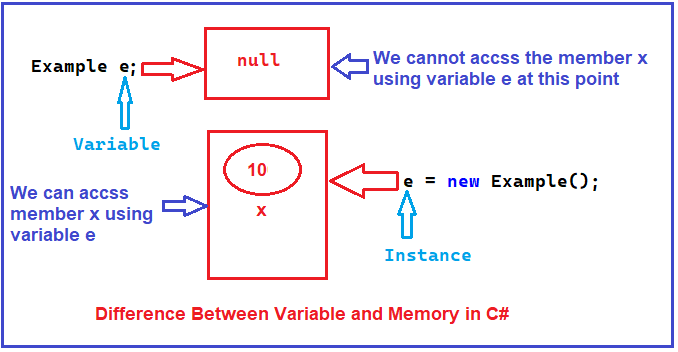Note: So, the point that you need to remember is until and unless you initialize the variable, the memory allocation will not be done. Once you initialize the variable using the new keyword, then the memory allocation will be done, and then only you can access the non-static members of the class. And instances are going to be created by using the new keyword only.

When we initialize a variable using the new keyword, then the variable becomes an instance. And you can declare and initialize the variable at the same line (using a single statement) or you can split the declaration and initialization into two statements as shown in the below image.##### Reference of a Class in C#:

Now, let us understand what is a reference for a class in C#. Please observe the following code. Here, I am creating two instances of the class Example i.e. e1 and e2. So, here, two times the memory will be allocated. One for e1 as we are using a new keyword and the other for e2 as again we are using the new keyword to initialize the instances. So, in the below examples, the memory will be allocated two times. And then we are printing the value of x using both instances.

```using System;
namespace VariablesDemo
{
internal class Example
{
int x = 10;
static void Main(string[] args)
{
Example e1 = new Example(); //e1 is Instance of class Example
Example e2 = new Example(); //e2 is Instance of class Example

Console.WriteLine(\$"e1.x: {e1.x} and e2.x: {e2.x}");
}
}
}
```

Now, when you run the above code, you will get the following output.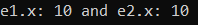Here, we have two copies of the variable x available in the memory. Let’s prove this. Let us modify the variable x to 50 of the e1 instance and then again let us print the value of x using both the instances as shown in the below example.

```using System;
namespace VariablesDemo
{
internal class Example
{
int x = 10;
static void Main(string[] args)
{
Example e1 = new Example(); //e1 is Instance of class Example
Example e2 = new Example(); //e2 is Instance of class Example

Console.WriteLine(\$"e1.x: {e1.x} and e2.x: {e2.x}");

e1.x = 50; //Modifying the x variable of e1 instance
Console.WriteLine(\$"e1.x: {e1.x} and e2.x {e2.x}");

}
}
}
```
###### Output: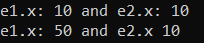As you can see in the above output, the x variable of the e1 instance is changed to 50 only. It will not change the x variable of the e2 instance. Now, let us modify the x variable of the e2 instance and then print the x value of both instances again as shown in the below example.

```using System;
namespace VariablesDemo
{
internal class Example
{
int x = 10;
static void Main(string[] args)
{
Example e1 = new Example(); //e1 is Instance of class Example
Example e2 = new Example(); //e2 is Instance of class Example

Console.WriteLine(\$"e1.x: {e1.x} and e2.x: {e2.x}");

e1.x = 50; //Modifying the x variable of e1 instance
Console.WriteLine(\$"e1.x: {e1.x} and e2.x {e2.x}");

e2.x = 150; //Modifying the x variable of e2 instance
Console.WriteLine(\$"e1.x: {e1.x} and e2.x {e2.x}");

}
}
}
```
###### Output: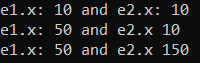As you can see in the above output, only the x variable of the e2 instance is changed to 150 and the x value of the e1 instance remains the same. So, this proves that we have two instances of the x variable available in the memory. For a better understanding, please have a look at the following diagram.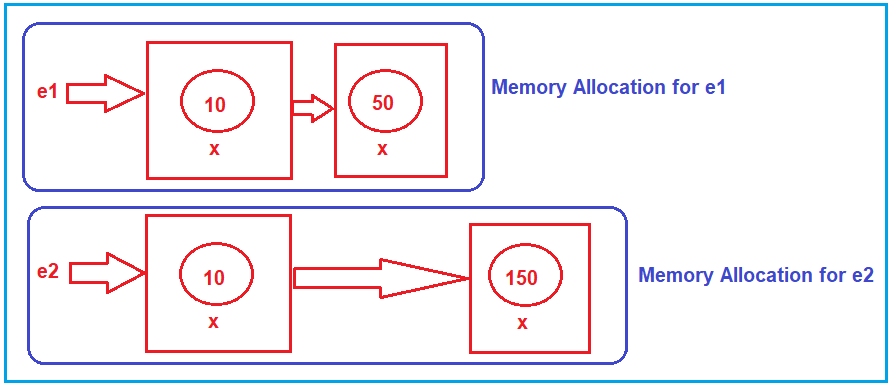Note: The point that you need to understand is that you can create any number of instances for your class, and for each instance, a separate memory allocation will be done. The memory allocation done for one instance is never going to be shared with other instances.

This is the beauty of Object-Oriented Programming or you can say it is the security of Object-Oriented Programming. Every instance is unique itself. The changes made in one instance will never reflect in other instances.

This is all about instances of a class. Now, let us talk about the References of a class in C#. Please have a look at the following code. Here, e1 is an instance of a class but e2 is a reference of a class. See, e2 is not initialized with the new keyword and hence it is not an instance. But e2 is initialized with an existing instance.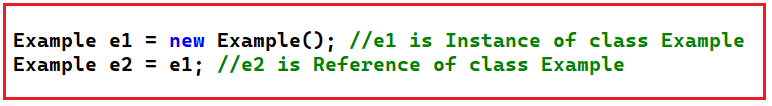So, the point that you need to remember is whenever a copy of the class is initialized with an existing instance, then it is called a reference of a class. Here, e2 is initialized with the existing instance e1 and hence e2 will be a reference of the class. Simply speaking now e2 is a pointer to e1. So, here e2 does not have a separate memory allocation rather it will point to the same memory allocation which is done by the e1 instance. That means both e1 and e2 are now consuming the same memory location. Let us prove this.

In the below example, e1 is an instance and e2 is a reference pointing to the e1 instance. Then we are modifying the x value to 50 using the e1 instance and if both are pointing to the same memory location, then the changes should also affect e2 also.

```using System;
namespace VariablesDemo
{
internal class Example
{
int x = 10;
static void Main(string[] args)
{
Example e1 = new Example(); //e1 is Instance of class Example
Example e2 = e1; //e2 is Reference of class Example

Console.WriteLine(\$"e1.x: {e1.x} and e2.x: {e2.x}");

e1.x = 50; //Modifying the x variable of e1 instance
Console.WriteLine(\$"e1.x: {e1.x} and e2.x {e2.x}");

}
}
}
```
###### Output: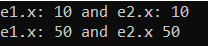As you can see in the above output, both e1 and e2 are printing the same value but we have made changes to the e1 instance but that changes should be affecting the e2. Now, let us modify the x variable to 150 of the e2 reference and see whether the changes affect the e1 instance or not.

```using System;
namespace VariablesDemo
{
internal class Example
{
int x = 10;
static void Main(string[] args)
{
Example e1 = new Example(); //e1 is Instance of class Example
Example e2 = e1; //e2 is Reference of class Example

Console.WriteLine(\$"e1.x: {e1.x} and e2.x: {e2.x}");

e1.x = 50; //Modifying the x variable of e1 instance
Console.WriteLine(\$"e1.x: {e1.x} and e2.x {e2.x}");

e2.x = 150; //Modifying the x variable of e2 reference
Console.WriteLine(\$"e1.x: {e1.x} and e2.x {e2.x}");

}
}
}
```
###### Output: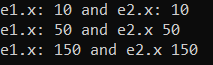As you can see the changes made in the e2 reference also affect the e1 instance. That means whether we made changes in e1 or e2, it will affect both. This is because both e1 and e2 are pointing to the same memory location. For a better understanding, please have a look at the following diagram.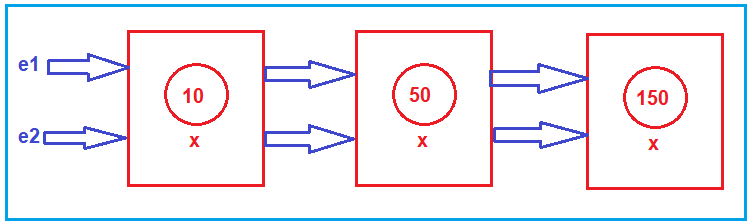Simply speaking reference of a class can be called a pointer to the instance and every modification we perform on the members using the instance will reflect when we access those members using references and vice versa is also true.

Note: Using variables, you cannot access the non-static members of a class, but using both instances or references, you can access the non-static members of a class. This is because the reference is initialized with an existing instance only and variables are never initialized.

Note: References do not have their own memory allocation, rather they will point to an existing memory. But references are used like instances.